# Multi-Stage Transistor Amplifiers

## Discrete Semiconductor Devices and Circuits

• #### Question 1

 Don’t just sit there! Build something!!

Learning to mathematically analyze circuits requires much study and practice. Typically, students practice by working through lots of sample problems and checking their answers against those provided by the textbook or the instructor. While this is good, there is a much better way.

You will learn much more by actually building and analyzing real circuits, letting your test equipment provide the “answers” instead of a book or another person. For successful circuit-building exercises, follow these steps:

1. Carefully measure and record all component values prior to circuit construction, choosing resistor values high enough to make damage to any active components unlikely.
2. Draw the schematic diagram for the circuit to be analyzed.
3. Carefully build this circuit on a breadboard or other convenient medium.
4. Check the accuracy of the circuit’s construction, following each wire to each connection point, and verifying these elements one-by-one on the diagram.
5. Mathematically analyze the circuit, solving for all voltage and current values.
6. Carefully measure all voltages and currents, to verify the accuracy of your analysis.
7. If there are any substantial errors (greater than a few percent), carefully check your circuit’s construction against the diagram, then carefully re-calculate the values and re-measure.

When students are first learning about semiconductor devices, and are most likely to damage them by making improper connections in their circuits, I recommend they experiment with large, high-wattage components (1N4001 rectifying diodes, TO-220 or TO-3 case power transistors, etc.), and using dry-cell battery power sources rather than a benchtop power supply. This decreases the likelihood of component damage.

As usual, avoid very high and very low resistor values, to avoid measurement errors caused by meter “loading” (on the high end) and to avoid transistor burnout (on the low end). I recommend resistors between 1 kΩ and 100 kΩ.

One way you can save time and reduce the possibility of error is to begin with a very simple circuit and incrementally add components to increase its complexity after each analysis, rather than building a whole new circuit for each practice problem. Another time-saving technique is to re-use the same components in a variety of different circuit configurations. This way, you won’t have to measure any component’s value more than once.

• #### Question 2

Why is it common for amplifier circuits to use multiple stages of transistors, rather than just one transistor (or two transistors in a push-pull circuit)? Describe some of the benefits of using multiple transistor stages.

• #### Question 3

Describe the function of each component in this two-stage amplifier circuit: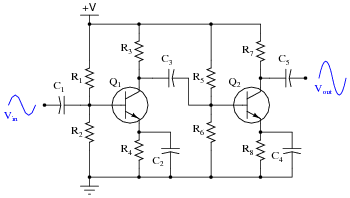Also, be prepared to explain what the effect of any one component’s failure (either open or shorted) will have on the output signal.

• #### Question 4

In some applications where transistors must amplify very high currents, bipolar transistors are paralleled together so that their current ratings add: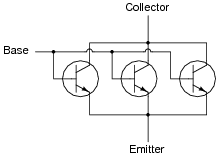However, if transistors are directly paralleled as shown, reliability problems may develop. A better way of “ganging” multiple transistors together is to connect a low-value swamping resistor to each emitter terminal:Explain what purpose these resistors serve in a paralleled transistor network. And what exactly does “swamping” mean, anyway?

• #### Question 5

In some applications where transistors must amplify very high currents, bipolar transistors are paralleled together so that their current ratings add. When this is done, it is a good idea to use swamping resistors at the transistor emitter connections to help ensure even balancing of currents: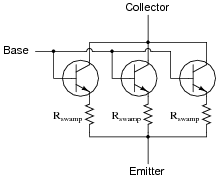However, if we use MOSFETs instead of BJTs, we do not have to use swamping resistors: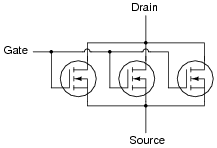Explain why MOSFETs do not require swamping resistors to help evenly distribute current, while BJTs do.

• #### Question 6

The first amplifier circuit shown here is direct-coupled, while the second is capacitively coupled.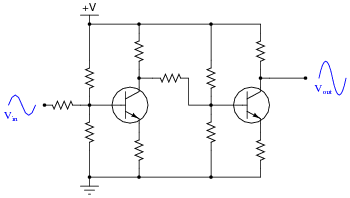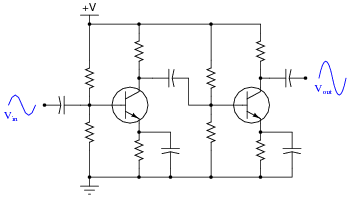Which of these two designs would be more suitable for use in a DC voltmeter circuit (amplifying a measured DC voltage)? What applications would the other amplifier design be suited for?

• #### Question 7

One of the problems with capacitively-coupled amplifier circuits is poor low-frequency response: as the input signal frequency decreases, all capacitive reactances increase, leading to a decreased voltage gain. One solution to this problem is the addition of a capacitor in the collector current path of the initial transistor stage: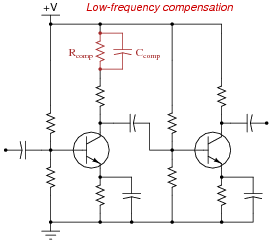Explain how the presence of this “compensating” capacitor helps to overcome the loss of gain normally experienced as a result of the other capacitors in the circuit.

• #### Question 8

This two-stage transistor amplifier circuit is transformer-coupled: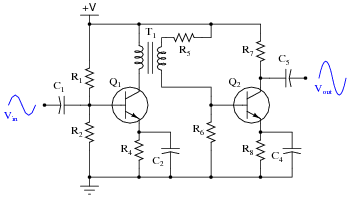What advantage(s) does a transformer-coupled amplifier have over circuits using other methods of coupling? Are there any disadvantages to using a transformer for signal coupling between transistor stages? Explain in detail.

• #### Question 9

Suppose two engineers were debating where to place a potentiometer in this audio amplifier circuit, to be used as a volume control: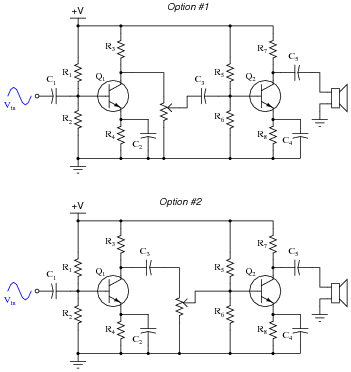Which option would be better, and why? What ill effects could result from locating the potentiometer in the wrong place in this circuit?

• #### Question 10

Radio-frequency amplifiers often use small inductors called peaking coils in the coupling circuitry between transistor stages. Describe the purpose of these inductors.

• #### Question 11

One design of push-pull audio amplifier uses two identical transistors and a center-tapped transformer to couple power to the load (usually a speaker, in an audio-frequency system):Unlike complementary-pair push-pull amplifier circuits, this circuit absolutely requires a preamplifier stage called a phase splitter, comprised here by transistor Q1 and resistors R3 and R4.

Explain what the purpose of the “phase splitter” circuit is, and why it is necessary to properly drive the power transistors Q2 and Q3.

Hint: determine the phase relationships of the voltage signals at the base, collector, and emitter terminals of transistor Q1, with respect to ground.

• #### Question 12

Examine this push-pull audio amplifier circuit: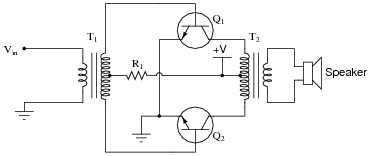How is phase splitting accomplished in this circuit?
What is the purpose of resistor R1?
What would happen if resistor R1 failed open?
What would happen if the wire connecting the base of transistor Q2 to the input transformer (T1) were to fail open?
• #### Question 13

The following amplifier circuit has a problem. Despite the presence of a strong input signal (as verified by an oscilloscope measurement at TP1), there is no sound coming from the speaker: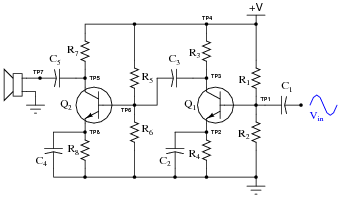Explain a logical, step-by-step approach to identifying the source of the problem, by taking voltage signal measurements. Remember, the more efficient your troubleshooting technique is (the fewer measurements taken), the better!

• #### Question 14

A common wideband transistor amplifier circuit is the cascode design, using common-emitter and common-base transistor stages:What advantage(s) does the cascode amplifier have over “normal” single- or multi-stage amplifier designs? What, specifically, makes it well suited for high-frequency applications, such as RF (Radio Frequency) signal amplifiers?

• #### Question 15

Suppose the following three-stage transistor amplifier were constructed:With no emitter swamping resistors anywhere in this circuit, the voltage gain of each stage is guaranteed to be large, but unstable as well. With three stages arranged like this, one feeding into the next, the final voltage gain will be very large, and very unstable.

However, if we add another resistor to the circuit (Rfeedback), something very interesting takes place. Suddenly, the amplifier circuit’s overall voltage gain is decreased, but the stability of this gain becomes much improved: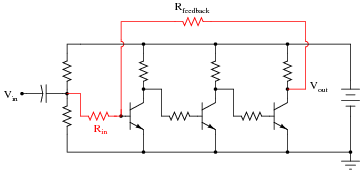Interestingly, the voltage gain of such a circuit will be nearly equal to the quotient of the two highlighted resistors, $$R_{feedback}$$ and $$R_{in}$$:

$$A_V \approx \frac{R_{feedback}}{R_{in}}$$

This approximation holds true for large variations in individual transistor gain (β) as well as temperature and other factors which would normally wreak havoc in the circuit with no feedback resistor in place.

Describe what role the feedback resistor plays in this circuit, and explain how the addition of negative feedback is an overall benefit to this circuit’s performance. Also, explain how you can tell this feedback is negative in nature (“degenerative”).

### Related Content

Published under the terms and conditions of the Creative Commons Attribution License•Tarikul Tuhin December 09, 2020